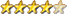Simple Maze using Truchet Tiles

Author Message
vegipeteGuruJoined: 29/01/2013
Posts: 971
 Posted: 12:01am 03 Apr 2021

Cool!

Here's a slight variation. I fudged the numbers slightly to get joined arcs, extended the loops to go past the screen edges, put a box around the perimeter and added a flood fill to colour a portion of the 'maze'. The red dot shows the start of the flood fill. Press any key for another image, [Esc] to quit.

Edit: added the option for corners instead of curves. Press "1" to change to corners, "0" to change to curves.

It would be handy to know how many pixels the flood fill command actually drew...

' Maze formed from Truchet Tiles
' This is basically the same algorithm as the famous
' Commodore Pet '10Print' one-line maze.
' Rev 1.0.0 William M Leue 4/1/2021
option default integer
option base 1

const TSIZE = 30
CORNERS = 0

do
cls
ncols = MM.HRES\TSIZE
nrows = MM.VRES\TSIZE
for row = 1 to nrows+1
y = (row-1)*TSIZE
for col = 1 to ncols+1
x = (col-1)*TSIZE
DrawTruchetTile x, y, rnd()
next col
next row
box 0,0,MM.HRES,MM.VRES
pixel fill ncols*TSIZE/2,nrows*TSIZE/2,rgb(blue)
circle ncols*TSIZE/2,nrows*TSIZE/2,TSIZE/4,0,1,,rgb(red)
do : k\$ = inkey\$ : loop until k\$ <> ""
if k\$ = "1" then CORNERS = 1
if k\$ = "0" then CORNERS = 0
loop until k\$ = chr\$(27)
end

sub DrawTruchetTile x, y, r as float
if CORNERS then
if r < 0.5 then
line x + TSIZE/2, y, x + TSIZE, y + TSIZE/2
line x, y + TSIZE/2, x + TSIZE/2, y + TSIZE
else
line x + TSIZE/2, y, x, y + TSIZE/2
line x + TSIZE, y + TSIZE/2, x + TSIZE/2, y + TSIZE
end if
else
if r < 0.5 then
arc x, y + TSIZE, TSIZE/2,, 0, 93
arc x + TSIZE, y, TSIZE/2,, 180, 273
else
arc x, y, TSIZE/2,, 87, 180
arc x + TSIZE, y + TSIZE, TSIZE/2,, -93, 0
end if
end if
end sub

Edited 2021-04-03 10:20 by vegipete